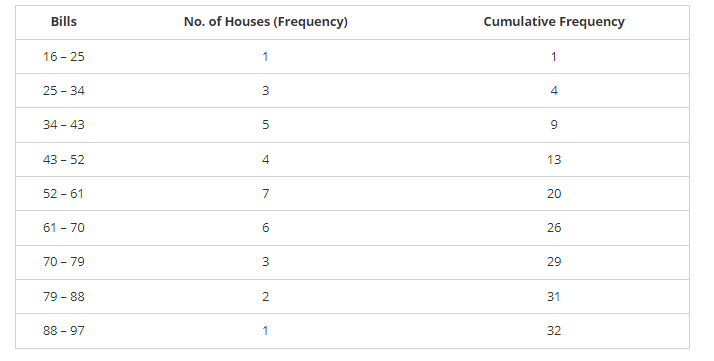# The water bills (in rupees) of 32 houses in a certain street for the period 1.198 to 31.398 are given below:

Question:

The water bills (in rupees) of 32 houses in a certain street for the period 1.198 to 31.398 are given below:

56, 43, 32, 38, 56, 24, 68, 85, 52, 47, 35, 58, 63, 74, 27, 84, 69, 35, 44, 75, 55, 30, 54, 65, 45, 67, 95, 72, 43, 65, 35, 69.

Tabulate the data and present the data as a cumulative frequency table using 70-79 as one of the class intervals.

Solution:

The minimum bill is Rs 24

The maximum bill is Rs 95

Range = Maximum bill-Minimum bill = 95 - 24 = 71

Given class interval is 70-79. So, Class size = 79 – 70 = 9

Therefore number of classes = Range/class size = 71/9 = 7.80

Number of classes = 8

The cumulative frequency distribution is as follows: